# An Active Fault-Tolerant Control Strategy for Current Sensors Failure for Induction Motor Drives Using a Single Observer for Currents Estimation and Axes Transformation

An Active Fault-Tolerant Control Strategy for Current Sensors Failure for Induction Motor Drives Using a Single Observer for Currents Estimation and Axes Transformation

Younes AzzougRemus Pusca Mohamed Sahraoui Abdelkarim Ammar Tarek Ameid Raphael Romary Antonio J. Marques Cardoso

Department of Electrical Engineering of the University of Biskra, Laboratory of Electrical Engineering of Biskra (LGEB), BP 145 RP, Biskra 07000, Algeria

Univ. Artois, UR 4025 LSEE F-62400 Bethune, France

Institute of Electrical and Electronics Engineering, Signal and Systems Laboratory, Avenue de l’indépendance, Boumerdes 35000, Algeria

CISE - Electromechatronic Systems Research Centre, Universidade da Beira Interior, Covilhã, Portugal

Corresponding Author Email:
younes_azzoug@ens.univ-artois.fr
Page:
467-474
|
DOI:
https://doi.org/10.18280/ejee.230606
31 January 2020
|
Accepted:
10 December 2021
|
Published:
31 December 2021
| Citation

OPEN ACCESS

Abstract:

This paper proposes a fault-tolerant control technique against current sensors failure in direct torque controlled induction motors drives, based on a new modification of Luenberger observer for currents estimation and axes transformation for vector rotation. Several important aspects are covered in the proposed algorithm, such as the detection of sensors failure, the isolation of faulty sensors, and the reconfiguration of the control system by a correct estimation. A logic circuit ensures fault detection by analyzing the residual signal between the measured and estimated quantities, while a single observer performs the task of estimating the line currents. In addition, a decision logic circuit isolates the erroneous signal and simultaneously selects the appropriate estimated current signal. An axes transformation ensures rotation from (a,b) to (α,β), which keeps a low-cost control using only two current sensors. The proposed scheme is tested on MATLAB/Simulink environment and experimentally validated in a laboratory prototype mainly containing a dS1104 card and 4 kW induction motor.

Keywords:

direct torque control, fault-tolerant control, fault detection, induction motor drive, current estimation

1. Introduction

Fault Tolerant Control (FTC) methods are crucial in variable speed drives used in critical and high-sensitivity applications, such as, space exploration, nuclear power plants, electric vehicles, air and rail transportation; in order to ensure system security, and uninterrupted operation, even with degraded performance, when an unexpected failure occurs in the variable speed drive. Consequently, a fault tolerant control strategy must be implemented to guarantee that faults are handled in such a way that there will be no damage.

Generally, variable speed Induction Motor (IM) drives need feedback information from a speed/position transducer, two or three current sensors and a dc-link voltage sensor at least , . Unfortunately, sensors are very prone to failures, and for this reason several research results have been published regarding sensors fault-tolerant control, as well as Fault Detection, Isolation, and Reconfiguration (FDIR) methods [3-10].

The study  presents a sensor FTC of an induction motor drive, based on the extended Kalman filter, and a reduced number of adaptive observers, to keep the operation of the system under the occurrence of sensors failures. In , a fault-tolerant vector control is proposed. A logic circuit is used to identify the faulty current sensor. The missing current information is replaced by an appropriate stator current, which value is calculated from algebraic equations and from the other measured healthy currents. However, this approach is not practical in the event of a successive failure in the different sensors. A fault-tolerant control scheme is proposed in Ref. , in which an adaptive fuzzy logic-based observer is used to generate residuals, which analysis allows the detection, and isolation of the faulty sensor. However, the dynamic response of this scheme for isolating defective sensors is too slow. In Ref. , it is proposed a sensor fault detection and isolation scheme for an IM drive, based on currents estimation and rotor resistance variations, which are then used in a decision unit for the identification and isolation of the faulty sensor. According to Yu et al. , a FTC technique against speed and current sensors failures in IM drives is proposed, and based on three adaptive observers with different inputs. However, this method is not suitable to achieve an efficient FTC in case of failures in two or three sensors. The results obtained by Yu et al.  offer a solution to the succession of faults in the different sensors but the number of the used adaptive observers is still three. Another type of FTC methods has been also presented in the literature, in which the sensors FTC algorithm changes the control according to the type of the faulty sensor, as proposed in Refs. [11-14]. A smooth FTC of IM drives with sensor failures is presented in the works [11, 12]. In the healthy state, the IM drive's operation is accomplished using direct torque control; indirect field-oriented control is then used when the dc-link voltage sensor fails, and v/f control is utilized during current sensors failure. The major drawback of this method, as reported by Tabbache et al. , is that these techniques are experimentally difficult to implement.

This paper proposes a direct torque fault-tolerant control strategy for the current sensor’s failure in induction motor drives. Some features are distinct from the other proposed methods. First, the estimation of stator currents is performed using a single observer. Then, the method proposed here allows the detection of faulty sensors even when several sensors fail successively, case in which a decision unit, based on a logic circuit, performs the task of reconfiguring and isolating the effects corresponding to the faulty sensor. Finally, the proposed technique can be reorganized in an adaptive way in the event of the loss of one or two sensors. The proposed scheme is simulated in MATLAB/Simulink environment, and experimentally implemented in a laboratory test bench under different operating conditions.

The remainder of the paper is organized into 5 sections. Section 2 analyses the direct torque control. Afterwards, section 3 describes the concept of the proposed FTC. Simulation and experimental results are presented and discussed in section 4. Finally, section 5 presents the main conclusion of the paper.

2. Direct Torque Control for the Induction Motor

The system equation below gives the induction motor model used in the present work.

$\left\{\begin{array}{c} \frac{d i_{s \alpha}}{d t}=-\left(\frac{R_{s}}{\sigma L_{s}}+\frac{R_{r}}{\sigma L_{r}}\right) i_{s \alpha}-\omega_{r} i_{s \beta} \\ +\frac{R_{s}}{\sigma L_{s} L_{r}} \psi_{s \alpha}+\frac{\omega_{r}}{\sigma L_{r}} \psi_{s \beta}+\frac{1}{\sigma L_{s}} V_{s \alpha} \\ \frac{d i_{s \beta}}{d t}=-\left(\frac{R_{s}}{\sigma L_{s}}+\frac{R_{r}}{\sigma L_{r}}\right) i_{s \beta}-\omega_{r} i_{s \alpha} \\ +\frac{R_{s}}{\sigma L_{s} L_{r}} \psi_{s \beta}+\frac{\omega_{r}}{\sigma L_{r}} \psi_{s \alpha}+\frac{1}{\sigma L_{s}} V_{s \beta} \\ \frac{d \psi_{s \alpha}}{d t}=V_{s \alpha}-R_{s} i_{s \alpha} \\ \frac{d \psi_{s \beta}}{d t}=V_{s \beta}-R_{s} i_{s \beta} \end{array}\right.$              (1)

where:

$V_{s \alpha}, V_{s \beta}:(\alpha, \beta)$ stator voltages,

$i_{s \alpha}, i_{s \beta}:(\alpha, \beta)$ stator currents,

$\psi_{s \alpha}, \psi_{s \beta}:(\alpha, \beta)$  stator fluxes,

$R_{s}, R_{r}$ : stator and rotor resistances,

$L_{s}, L_{r}$: stator and rotor inductances,

$\omega_{r}$ : rotor angular speed.

$\sigma=1-\frac{M^{2}}{L_{s} L_{r}}$ , given that M is the mutual inductance.

The electromagnetic torque is expressed by the following equation:

$T_{e}=p\left(\psi_{s \alpha} i_{s \beta}+\psi_{s \beta} i_{s \alpha}\right)$          (2)

The stator flux components are given in the system equation below:

$\left\{\begin{array}{l} \psi_{s \alpha}=\int\left(V_{s \alpha}-R_{s} i_{s \alpha}\right) d t \\ \psi_{s \beta}=\int\left(V_{s \beta}-R_{s} i_{s \beta}\right) d t \end{array}\right.$          (3)

The Direct Torque Control (DTC) basic idea is to estimate flux and torque instantaneous values only from the stator variables. The estimated torque is compared with the reference one given by the speed controller (PI controller in the present work), the resulting torque error signal is sent to a hysteresis controller (three-levels hysteresis controller is adopted in the present work) to get a control signal $\mathrm{C}_{\mathrm{Te}}$, which fed a switching look-up table. Likewise, to the estimated flux, which is compared with fixed value of a stator flux magnitude, the resulting flux error signal is fed an hysterisis controller (two-levels hysterisis controller is adopted in the present work) to get a control signal $\mathrm{C}_{\mathrm{flx}}$ for the switchin look-up table. This latter uses flux and torque control signals in order to generate three control signals $\mathrm{S}_{\mathrm{A}}, \mathrm{S}_{\mathrm{B}} \text { and } \mathrm{S}_{\mathrm{C}}$ for the three-phase inverter (see Figure 4).

3. Concept of the Proposed Fault-Tolerant Control

In the proposed fault-tolerant direct torque control, two current sensors in phase-a, and phase-b are used in addition to a dc-link voltage sensor, and an incremental encoder. Furthermore, using the control signals SA, SB and SC, a voltage synthesizer constructs the three-phase stator voltages applied to the machine from the voltage measured in the dc-link.

3.1 Stator currents estimation

In order to estimate the line currents, a modified adaptive observer is used, which gives high-performance estimation stator currents, based on the IM model, which is described by the following state equation:

$\left\{\begin{array}{c} \dot{X}=A\left(\omega_{r}\right) X+B U \\ Y=C X \end{array}\right.$         (4)

here:

$\begin{gathered} X=\left[\begin{array}{lll} i_{s \alpha} & i_{s \beta} & \psi_{r \alpha} \psi_{r \beta} \end{array}\right]^{T} ; Y=\left[\begin{array}{ll} i_{s \alpha} & i_{s \beta} \end{array}\right]^{T} \\ U=\left[\begin{array}{l} V_{s \alpha} V_{s \beta} \end{array}\right]^{T} \end{gathered}$

and:

$\begin{gathered} A=\left[\begin{array}{cccc} a_{1} & 0 & a_{2} & \omega_{r} a_{3} \\ 0 & a_{1} & -\omega_{r} a_{3} & a_{2} \\ a_{4} & 0 & a_{5} & -\omega_{r} \\ 0 & a_{4} & \omega_{r} & a_{5} \end{array}\right] ; B=\left[\begin{array}{cc} \frac{1}{\sigma L_{s}} & 0 \\ 0 & \frac{1}{\sigma L_{s}} \\ 0 & 0 \\ 0 & 0 \end{array}\right] ; \\ C=\left[\begin{array}{llll} 1 & 0 & 0 & 0 \\ 0 & 1 & 0 & 0 \end{array}\right] \end{gathered}$

as well as:

$\begin{gathered} a_{1}=-\left(\frac{1}{\tau_{s} \sigma}+\frac{(1-\sigma)}{\tau_{r} \sigma}\right) ; \\ a_{2}=\frac{M}{\sigma L_{s} L_{r} \tau_{r}} ; a_{3}=\frac{M}{\sigma L_{s} L_{r}} ; a_{4}=\frac{M}{\tau_{r}} ; \\ a_{5}=\frac{1}{\tau_{r}} ; \tau_{s}=\frac{L_{s}}{R_{s}} ; \tau_{r}=\frac{L_{r}}{R_{r}} ; \end{gathered}$

The idea of the proposed stator currents estimator is based on the general theory of Luenberger observer , which allows the estimation of variable or unknown parameters of a system. The equation of the Luenberger observer is expressed in (5), where the symbol “^” denotes the estimated values:

$\left\{\begin{array}{c} \dot{\hat{X}}=A\left(\omega_{r}\right) \hat{X}+B U+K \xi \\ \hat{Y}=C \hat{X} \end{array}\right.$         (5)

where:

$\begin{gathered} \hat{X}=\left[\widehat{l_{s \alpha}} \widehat{l_{s \beta}} \widehat{\psi_{r \alpha}} \widehat{\psi_{r \beta}}\right]^{T} ; \hat{Y}=\left[\widehat{l_{s \alpha}} \widehat{l_{s \beta}}\right]^{T} ; \\ \xi=\left[i_{s \alpha}-\widehat{l_{s \alpha}} i_{s \beta}-\widehat{l_{s \beta}}\right]^{T} \end{gathered}$

Since there is no information about the measured currents, the vector ξ becomes:

$\xi=\left[-\widehat{l_{s \alpha}}-\widehat{l_{s \beta}}\right]^{T}$

The estimation is performed throw the conservation of the state model (4) of the IM with the gain matrix K. This matrix ensures the convergence of the observer, and has been determined by the classical pole placement procedure. Then, replacing the adaptive mechanism by the measured rotational speed, as well as, feeding the observer with the stator voltages provided by the voltage synthesizer. This gives the stator currents estimation, as illustrated in Figure 1.

## 1.png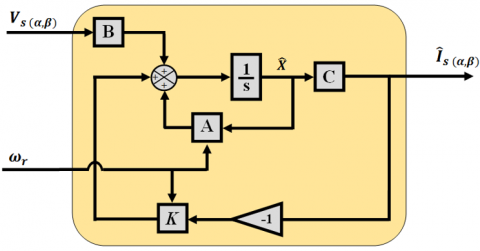Figure 1. Proposed stator currents estimator

Considering the following system of equations, that present the current observer model in the complex format, the gain matrix K is defined as follows:

$\left\{\begin{array}{c} \dot{\bar{X}}=\bar{A} \hat{X}+B \bar{V}_{s}+\bar{K} \xi \\ \hat{Y}=C \hat{\bar{X}} \end{array}\right.$        (6)

The determination of the gain matrix K uses the conventional pole placement procedure, through the imposition of the observer's poles and consequently of its dynamics [17-20].

The characteristic equation of the observer is as follow:

$G(s)=\operatorname{det}(s I-(\bar{A}-\bar{K} C))$         (7)

Developing the different matrices A, K and C knowing that $\bar{K}=\left[\begin{array}{l} K^{\prime} \\ K^{\prime \prime} \end{array}\right]$ the following equation is obtained:

\begin{aligned} G(s)=s^{2}+\left(\frac{1}{\sigma T_{s}}\right.&\left.+\frac{1}{\sigma T_{r}}-j \omega_{r}+K^{\prime}\right) s \\ &+\left(\frac{1}{T_{r}}-j \omega_{r}\right)\left\{\left(\frac{1}{\sigma T_{s}}+\frac{1}{\sigma T_{r}}\right)+K^{\prime}\right\} \\ &+\left(\frac{M}{T_{r}}-K^{\prime \prime}\right)\left(\frac{M}{\sigma L_{s} L_{r}}\right)\left(\frac{1}{T_{r}}-j \omega_{r}\right) \end{aligned}         (8)

where, K' and K'' are complex gains.

The dynamic equation of the observer is defined as:

\begin{aligned} H(s)=s^{2}+l\left(\frac{1}{\sigma T_{s}}\right.&\left.+\frac{1}{\sigma T_{r}}-j \omega_{r}\right) s \\ &+l^{2}\left(\frac{1}{T_{r}}-j \omega_{r}\right)\left\{\left(\frac{1}{\sigma T_{s}}+\frac{1}{\sigma T_{r}}\right)\right\} \\ &+\left(\frac{M}{T_{r}}\right)\left(\frac{M}{\sigma L_{s} L_{r}}\right)\left(\frac{1}{T_{r}}-j \omega_{r}\right) \end{aligned}             (9)

where, $l$ is the proportionality constant, slightly bigger than the unit ($l$=1.004).

Eqns. (8) and (9) are in the form of the following equation:

$H P(s)=s^{2}+2 \varepsilon \omega_{n} s+\omega_{n}^{2}$          (10)

The comparison of Eqns. (8) and (9) gives for the expressions of K' and K'':

$\left\{\begin{array}{c} K^{\prime}=(l-1)\left(\frac{1}{\sigma T_{s}}+\frac{1}{\sigma T_{r}}-j \omega_{r}\right) \\ K^{\prime \prime}=(l-1)\left[\begin{array}{c} \left\{\left[\frac{1}{\sigma T_{s}}+\frac{1}{\sigma T_{r}}\right] \frac{\sigma L_{s} M}{L_{r}}-\frac{M}{T_{r}}\right\} \\ (l+1)-\frac{\sigma L_{s} M}{L_{r}}\left[\frac{1}{\sigma T_{s}}+\frac{1}{\sigma T_{r}}\right] \\ +j \omega_{r} \frac{\sigma L_{s} M}{L_{r}} \end{array}\right] \end{array}\right.$           (11)

To get the gain matrix K it is supposed that:

$\left\{\begin{array}{l} K^{\prime}=K_{1}+j K_{2} \\ K^{\prime \prime}=K_{3}+j K_{4} \end{array}\right.$                 (12)

So,

$\begin{gathered} K_{1}=(l-1)\left(\frac{1}{\sigma \tau_{s}}+\frac{1}{\sigma \tau_{r}}\right) \\ K_{2}=-(l-1) \omega_{r} \\ K_{3}=\left(l^{2}-1\right)\left[\left(\frac{1}{\sigma \tau_{s}}+\frac{1}{\sigma \tau_{r}}\right) \frac{\sigma L_{s} M}{L_{r}}-\frac{M}{\tau_{r}}\right] \\ +\frac{\sigma L_{s} M}{L_{r}}\left(\frac{1}{\sigma \tau_{s}}+\frac{1}{\sigma \tau_{r}}\right)(l-1) \\ K_{4}=-(l-1) \frac{\sigma L_{s} M}{L_{r}} \omega_{r} \end{gathered}$

According to the anti-symmetry of matrix A, the gain matrix K is set as follows:

$K=\left[\begin{array}{cccc} K_{1} & K_{2} & K_{3} & K_{4} \\ -K_{2} & K_{1} & -K_{4} & K_{3} \end{array}\right]^{T}$

3.1.1 Currents estimator stability

Considering the following equations:

$\text { Induction motor model: } \dot{X}=A X+B U$          (13)

and

$\text { Observer model: } \dot{\hat{X}}=A \hat{X}+B U+K \xi$                (14)

The estimated stator currents error $\left(e=I_{S}-\widehat{I}_{S}\right)$  is the deference between the observer and the induction motor models, so:

$\dot{e}=(A-K C) e$          (15)

Considering the Lyapunov function below:

$S=e^{T} e+\frac{\left(\Delta \omega_{\mathrm{r}}\right)^{2}}{\lambda}$          (16)

Its time derivative is:

$\frac{d S}{d t}=\left(\frac{d e^{T}}{d t}\right) e+\left(\frac{d e}{d t}\right) e^{T}+\frac{1}{\lambda} \frac{d}{d t}\left(\Delta \omega_{r}\right)^{2}$         (17)

Knowing that $\Delta \omega_{r}=0$ , then:

$\frac{d S}{d t}=\left(\frac{d e^{T}}{d t}\right) e+\left(\frac{d e}{d t}\right) e^{T}$          (18)

Replacing Eq. (15) in Eq. (18) gives:

$\frac{d S}{d t}=e\left\{(A-K C)^{T}+(A-K C)\right\} e^{T}$            (19)

A sufficient condition for this estimator stability is that Eq. (19) is equal to zero, and indeed, this equation is always equal to zero.

3.2 Fault detection, isolation and system reconfiguration

The logic-circuit presented in Figure 2 is intended to ensure the fault detection, isolation, and system reconfiguration. The detection of failures is performed by analyzing the residual signal between the measured and estimated quantities, passing through a Low Pass Filter (LFP) to extract the useful signal that will be compared to a well-defined threshold (Th=0.8), then a proper estimation is used for the isolation and reconfiguration of the system.

## 2.png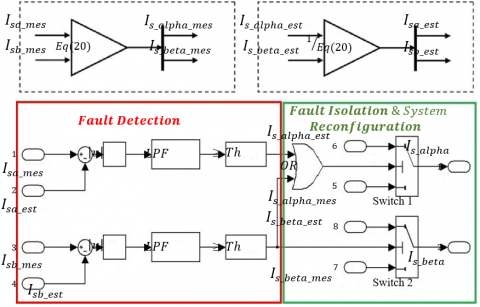Figure 2. Fault detection, isolation and system reconfiguration scheme

As mentioned previously, two current sensors (in phase-a and phase-b) were used in the proposed method for measuring a and b line currents. The axis transformation presented in Figure 3 developed by Chakraborty and Verma  and used in both [22, 23], which is also used in the proposed FTC, in order to have the component currents $(\alpha, \beta)$ using only the measurements of (a, b) components.

## 3.png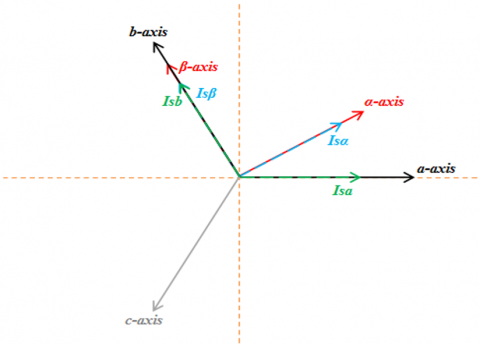Figure 3. Axis transformation model

As shown in Figure 3, the β-axis is aligned with the b-axis. Considering the equation of transformation from (a, b) to $(\alpha, \beta)$, which is given in (20), it is clear that $i_{s \alpha}$ depends on the two-phase currents $I_{s a} \text { and } I_{s b}$, whereas the current $i_{s \beta}$ depends only on the current $I_{s b}$. Therefore, if the phase-a current sensor becomes faulty, only $i_{S \alpha}$ that will be affected. But, if the phase-b current sensor becomes faulty, both currents $i_{s \alpha} \text { and } i_{s \beta}$ will be affected.

$\left[\begin{array}{l} i_{s \alpha} \\ i_{s \beta} \end{array}\right]=\left[\begin{array}{cc} 2 / \sqrt{3} & 1 / \sqrt{3} \\ 0 & 1 \end{array}\right]\left[\begin{array}{l} I_{s a} \\ I_{s b} \end{array}\right]$          (20)

So, the faulty measured current components $(\alpha, \beta)$ must be replaced by the corresponding estimated currents. As a consequence, Table 1 shows the identification of the faulty sensors and the correct components of the estimated currents that will replace the erroneous components.

Table 1. Faulty sensor identification and correct component selection

 Sensor-a Sensor-b Za Zb OR Proper selected components Healthy Healthy 0 0 0 $i_{s \alpha \text { mes }} \text { and } i_{s \beta \text { mes }}$ Faulty Healthy 1 0 1 $i_{s \alpha e s t} \text { and } i_{s \beta \text { mes }}$ Healthy Faulty 0 1 1 $i_{s \alpha \text { est }} \text { and } i_{s \beta \text { est }}$ Faulty Faulty 1 1 1 $i_{s \alpha e s t} \text { and } i_{s \beta e s t}$

As presented in Figure 2 and defined in Table I, it appears that in the case of sensor-a failure, fault detection part of the logic circuit generates an impulse Za=1, whereas, in the case of sensor-b failure, fault detection logic circuit generates an impulse Zb=1. Once the faulty sensor is identified, it is isolated, and the system is reconfigured, using the fault isolation and system reconfiguration part of the logic circuit. The logic gate OR output is 1 when any one of its inputs Za or Zb or both is 1 due to the effect of the failure of sensor-b on $i_{s \alpha}$ current. As a consequence, in the case of sensor-b failure, $i_{s \alpha \text { mes }}$ is isolated and replaced by isa est.

4. Simulation and Experimental Results

The proposed current sensor direct torque fault-tolerant control for induction motor drive is simulated in Matlab\Simulink environment using 4 kW induction motor parameters (see the Appendix). Figure 4 presents the block diagram of the proposed method.

## 4.png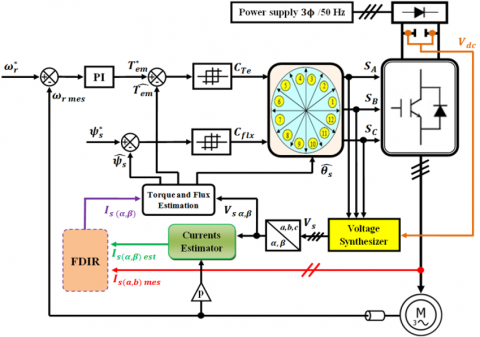Figure 4. Block diagram of the proposed FTC

Figure 5 displays the laboratory test bench in which the proposed control technique has been implemented and tested experimentally, which is composed mainly of a 4 kW three-phase IM whose parameters are the same used in simulation model, dS1104 card, two current sensors, and dc-voltage sensor.

## 5.png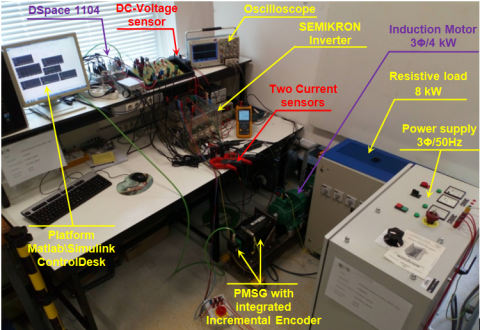Figure 5. Laboratory experimental test bench

All simulation and experimental tests are performed at 1000 rpm with 20 N.m load torque.

4.1 Test of the proposed FTC when sensor-a becomes faulty and sensor-b remains healthy

Figures 6 and 7 show simulation and experimental results obtained respectively, applying the proposed FTC. The drive was started with healthy current sensors in both simulation and experimentation. At t=1 s in simulation test and t=10.6 s in experimental test, current sensor-a fails. As a result and corresponding to the used axis transformation equation (6), isα mes becomes faulty such is depicted in Figures 6(a) and 7(a), and isβ mes remains healthy as can be seen in Figures 6(b) and 7(b). At the moment of failure occurrence, Za sensor-a state indicator becomes 1, judging sensor-a faulty, but Zb sensor-b state indicator remains 0, affirming that sensor-b is healthy, as illustrated in Figures 6(c) and 7(c). As a consequence of Za=1, switch-a switches to input 1 to replace the erroneous measured current by the estimated one as explained previously in Table 1. Figures 6(d), 7(d) and 6(e), 7(e) illustrate respectively the high-performance speed tracking of the IM drive, and the uninterrupted fulfillment of electromagnetic torque requirement under both healthy and faulty conditions, and Figures 6(f), 7(f) depict stator flux circular trajectory.

## 6.png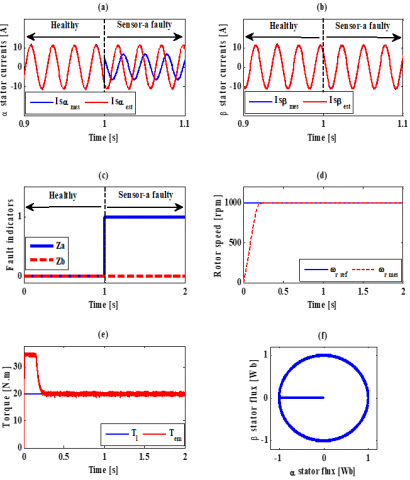Figure 6. Simulation results when sensor-a is faulty and sensor-b remains healthy: (a) i currents, (b) i currents, (c) fault indicator, (d) reference and actual speeds, (e) load and electromagnetic torque, and (f) stator flux trajectory

## 7.png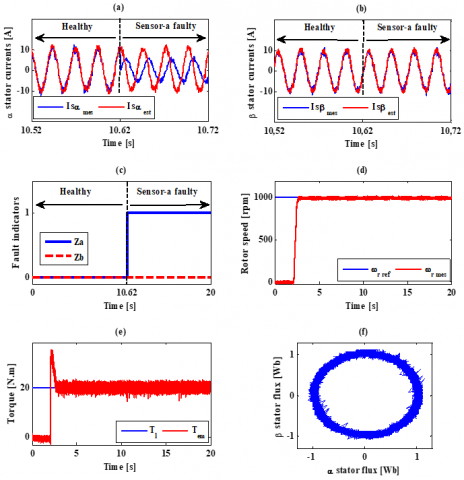Figure 7. Experimental results when sensor-a is faulty and sensor-b remains healthy: (a) i currents, (b) i currents, (c) fault indicator, (d) reference and actual speeds, (e) load and electromagnetic torque, and (f) stator flux trajectory

4.2 Test of the proposed FTC when sensor-b becomes faulty and sensor-a remains healthy

Figures 8 and 9 give another test of the suggested FTC method with simulation and experimentation respectively, where a failure in sensor-b is performed, whereas sensor-a remains healthy. Simulation and experimental tests were started in healthy conditions until t=1 s for simulation test and t=9.8 s for experimental test, where sensor-b becomes faulty. Considering Figures 8(a) and 9(a), it can be seen that $i_{s \alpha \text { mes }}$ is slightly affected by the failure of sensor-b, while $i_{s \beta \text { mes }}$ is significantly affected, which is explained previously in Eq. (6). The fault detection algorithm reacts quickly by generating an impulse Zb=1, indicating that sensor-b is faulty, unlike Za which remains at 0, meaning that sensor-a gives proper measurement. At this moment, switch-b switches to input 1 to replace $i_{s \beta \text { mes }}$ by $i_{s \beta \text { est }}$, and according to the output of the gate OR (see Table 1) switch-a switches to input 1 to replace $i_{s \alpha \text { mes }}$ by $i_{s \alpha \text { est }}$. Figures 8(c) and 9(c) demonstrate sensor state indicators Za and Zb. Figures 8(d) for simulation and 9(d) for experimentation show the speed tracking performance of the IM drive before and after the failure occurrence.

## 8.png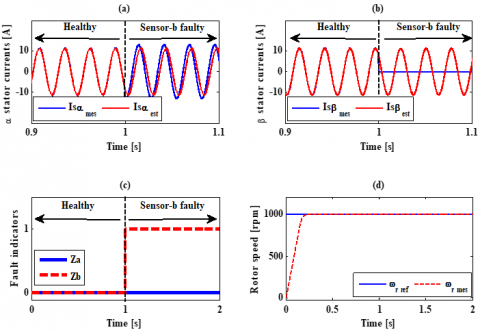Figure 8. Simulation results when sensor-b is faulty and sensor-a remains healthy: (a) i currents, (b) i currents, (c) fault indicator, and (d) reference and actual speeds

## 9.png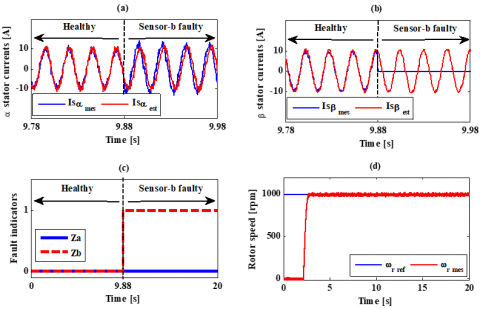Figure 9. Experimental results when sensor-b is faulty and sensor-a remains healthy: (a) i currents, (b) i currents, (c) fault indicator, and (d) reference and actual speeds

4.3 Test of the proposed FTC when both sensor-a and sensor-b become faulty

Figures 10 and 11 illustrate respectively the simulation and experimentation results obtained by applying the proposed FTC when the both sensors fail. The sensor-a failure is simulated at t=1s, while the sensor-b fault is simulated at t=1.1 s as shown in the Figures 10(a) and 10(b). In the experimental test, sensor-a failure is appeared at t=8.75 s, while sensor-b failure is appeared at t=14.35 s as can be seen in Figures 11(a) and 11(b). While, Figures 10(c) and 11(c) show the fault indicator signals (Za for sensor-a and Zb for sensor-b). When the defect is suddenly introduced in the sensor-a, the fault detection mechanism has reacted quickly by generating a Za index equal to 1, indicating that sensor-a is faulty. Based on Eq. (6), the current isβ mes will not be affected, which is visible in Figures 10(b) and 11(b), where the current isβ mes remains healthy from 0 to 1.1 s in simulation and until 14.34 s in experimentation. According to Table 1, the selected currents to isolate the faulty measurement are: isα est and isβ mes. After 1.1s in simulation and 14.35s in experimentation, a fault has been introduced in sensor-b, at this moment, the fault indicator Zb becomes 1, confirming sensor-b failure. Referring to equation (6), we can see that the currents i and i both depend on the state of the measurment of sensor-b, which is confirmed in Figures 10(a) and 11(a) where the degree of severity of the fault in the current isα mes is increased. Therefore, the isolation and reconfiguration algorithm choose isα est and isβ est (see Table 1).

## 11.png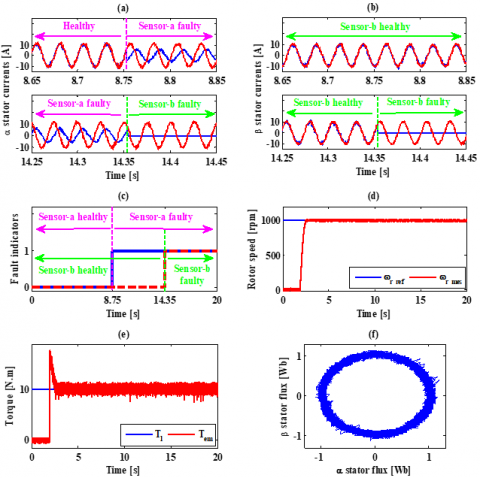Figure 10. Simulation results when both sensor-a and sensor-b become faulty: (a) i currents, (b) i currents, (c) fault indicator, (d) reference and actual speeds, (e) load and electromagnetic torque, and (f) stotor flux trajectory

## 11.pngFigure 11. Experimental results when both sensor-a and sensor-b become faulty: (a) i currents, (b) i currents, (c) fault indicator, (d) reference and actual speeds, (e) load and electromagnetic torque, and (f) stotor flux trajectory

Figures 10(d), 11(d) and 10(e), 11(e) clearly show that speed and torque maintain their performance and remain uninterrupted, even under the worst possible conditions, when the both current sensors fail. In addition, Figures 10(f) and 11(f) show the constant estimate of stator flux.

5. Conclusion

This paper presents a direct torque fault-tolerant control diagram for current sensors failures in the induction motor drives. For the identification of a sensor failure, a logic circuit is used in the phases where the current sensors are located, which is able to detect the failures of the used current sensors. A current estimator is used to estimate the stator currents. Immediately after the failure of the current sensor, a decision logic circuit automatically selects the correct current signal to maintain the operation of the system. Simulation and experimental results show the effectiveness of the proposed method, where the fault detection is accomplished with fast dynamics, and the drive is performing successfully, without compromising the control system.

Acknowledgment

This work has been achieved within the framework of CE2I project (Convertisseur d’Energie Intégré Intelligent). CE2I is co-ﬁnanced by European Union with the ﬁnancial support of the European Regional Development Fund (ERDF), French State and the French Region of Hauts-de-France.

As well, this work was supported by the European Regional Development Fund (ERDF) through the Operational Program for Competitiveness and Internationalization - COMPETE 2020, and also by National Funds through the Portuguese Foundation for Science and Technology (FCT), under Project POCI-01-0145-FEDER-029494 and Project UID/EEA/04131/2013.

Nomenclature
 IM Induction Motor DTC Direct Torque Control FTC Fault Tolerant Control FDIR Fault Detection, Isolation and Reconfiguration PMSG Permanent Magnet Synchronous Generator AC Alternative Current DC Direct Current Vdc DC-link voltage Vs Three phases stator voltages $I_{a}, I_{b}, I_{c}$ Three phases stator currents $V_{s \alpha}, V_{s \beta}$ $(\alpha, \beta)$ axis stator voltages $I_{s \alpha}, I_{s \beta}$ $(\alpha, \beta)$  axis stator currents $\varphi_{s \alpha}, \varphi_{s \beta}$ $(\alpha, \beta)$  axis stator fluxes $\varphi_{r \alpha}, \varphi_{r \beta}$ $(\alpha, \beta)$  axis rotor fluxes $\varphi_{r}$ Rotor flux magnitude $\omega_{S}$ Synchronous speed $\omega_{r}$ Rotor angular speed $\omega_{e}$ Electrical angular speed $\Omega_{r}$ Mechanical speed $T_{e}, T_{l}$ Electromagnetic and load torques
Appendix

The used induction motor specifications and parameters:

 Specifications Parameters Nominal power [kW] 4 Rs [Ω] 1.5 Nominal voltage [V] 400 Rr [Ω] 2.03 Nominal current [A] 9.2 Ls [H] 0.36 Frequency [Hz] 50 Lr [H] 0.36 Number of pole pairs 2 M [H] 0.35 Nominal speed [rpm] 1415 J [Kg.m2] 0.024 f [Nm.s.rad-1] 0.002
References

 Berriri, H., Naouar, M.W., Slama-Belkhodja, I. (2011). Easy and fast sensor fault detection and isolation algorithm for electrical drives. IEEE Transactions on Power Electronics, 27(2): 490-499. https://doi.org/10.1109/TPEL.2011.2140333

 Yu, Y., Chen, X., Dong, Z. (2017). Current sensorless direct predictive control for induction motor drives. In 2017 IEEE Transportation Electrification Conference and Expo, Asia-Pacific (ITEC Asia-Pacific) pp. 1-6. https://doi.org/10.1109/ITEC-AP.2017.8080975

 Zhang, X., Foo, G., Don Vilathgamuwa, M., Tseng, K.J., Bhangu, B.S., Gajanayake, C. (2013). Sensor fault detection, isolation and system reconfiguration based on extended Kalman filter for induction motor drives. IET Electric Power Applications, 7(7): 607-617. https://doi.org/10.1049/iet-epa.2012.0308

 Dybkowski, M., Klimkowski, K. (2016). Stator current sensor fault detection and isolation for vector controlled induction motor drive. In 2016 IEEE International Power Electronics and Motion Control Conference (PEMC), pp. 1097-1102. https://doi.org/10.1109/EPEPEMC.2016.7752147

 Lee, K.S., Ryu, J.S. (2003). Instrument fault detection and compensation scheme for direct torque controlled induction motor drives. IEE Proceedings-Control Theory and Applications, 150(4): 376-382. https://doi.org/10.1049/ip-cta:20030596

 Najafabadi, T.A., Salmasi, F.R., Jabehdar-Maralani, P. (2010). Detection and isolation of speed-, DC-link voltage-, and current-sensor faults based on an adaptive observer in induction-motor drives. IEEE Transactions on Industrial Electronics, 58(5): 1662-1672. https://doi.org/10.1109/TIE.2010.2055775

 Yu, Y., Wang, Z., Xu, D., Zhou, T., Xu, R. (2014). Speed and current sensor fault detection and isolation based on adaptive observers for IM drives. Journal of Power Electronics, 14(5): 967-979. https://doi.org/10.6113/JPE.2014.14.5.967

 Yu, Y., Zhao, Y., Wang, B., Huang, X., Xu, D. (2017). Current sensor fault diagnosis and tolerant control for VSI-based induction motor drives. IEEE Transactions on Power Electronics, 33(5): 4238-4248. https://doi.org/10.1109/TPEL.2017.2713482

 Mendes, A.M., Cardoso, A.M. (2006). Fault-tolerant operating strategies applied to three-phase induction-motor drives. IEEE Transactions on Industrial Electronics, 53(6): 1807-1817. https://doi.org/10.1109/TIE.2006.885137

 Azzoug, Y., Menacer, A., Pusca, R., Romary, R., Ameid, T., Ammar, A. (2018). Fault tolerant control for speed sensor failure in induction motor drive based on direct torque control and adaptive stator flux observer. In 2018 International Conference on Applied and Theoretical Electricity (ICATE), pp. 1-6. https://doi.org/10.1109/ICATE.2018.8551478

 Stettenbenz, M., Liu, Y., Bazzi, A. (2015). Smooth switching controllers for reliable induction motor drive operation after sensor failures. In 2015 IEEE Applied Power Electronics Conference and Exposition (APEC), pp. 2407-2411. https://doi.org/10.1109/APEC.2015.7104685

 Liu, Y., Stettenbenz, M., Bazzi, A.M. (2018). Smooth fault-tolerant control of induction motor drives with sensor failures. IEEE Transactions on Power Electronics, 34(4): 3544-3552. https://doi.org/10.1109/TPEL.2018.2848964

 Sepe, R.B., Morrison, C., Miller, J.M. (2003). Fault-tolerant operation of induction motor drives with automatic controller reconfiguration. Practical Failure Analysis, 3(1): 64-70. https://doi.org/10.1109/IEMDC.2001.939291

 Aguilera, F., Pablo, M., De Angelo, C.H. (2015). A fault tolerant system for current sensors in induction motor drives. In 2015 XVI Workshop on Information Processing and Control (RPIC), pp. 1-7. https://doi.org/10.1109/RPIC.2015.7497178

 Tabbache, B., Rizoug, N., Benbouzid, M.E.H., Kheloui, A. (2012). A control reconfiguration strategy for post-sensor FTC in induction motor-based EVs. IEEE Transactions on Vehicular Technology, 62(3): 965-971. https://doi.org/10.1109/TVT.2012.2232325

 Luenberger, D. (1971). An introduction to observers. IEEE Transactions on Automatic Control, 16(6): 596-602. https://doi.org/10.1109/TAC.1971.1099826

 Keller, H. (1987). Non-linear observer design by transformation into a generalized observer canonical form. International Journal of Control, 46(6): 1915-1930. https://doi.org/10.1080/00207178708934024

 Leu, Y.G., Lee, T.T., Wang, W.Y. (1999). Observer-based adaptive fuzzy-neural control for unknown nonlinear dynamical systems. IEEE Transactions on Systems, Man, and Cybernetics, Part B (Cybernetics), 29(5): 583-591. https://doi.org/10.1109/3477.790441

 Kubota, H., Sato, I., Tamura, Y., Matsuse, K., Ohta, H., Hori, Y. (2002). Regenerating-mode low-speed operation of sensorless induction motor drive with adaptive observer. IEEE Transactions on Industry Applications, 38(4): 1081-1086. https://doi.org/10.1109/TIA.2002.800575

 Miklosovic, R., Radke, A., Gao, Z. (2006). Discrete implementation and generalization of the extended state observer. In 2006 American Control Conference, pp. 6-pp. https://doi.org/10.1109/ACC.2006.1656547

 Chakraborty, C., Verma, V. (2014). Speed and current sensor fault detection and isolation technique for induction motor drive using axes transformation. IEEE Transactions on Industrial Electronics, 62(3): 1943-1954. https://doi.org/10.1109/TIE.2014.2345337

 Manohar, M., Das, S., Kumar, R. (2017). A robust current sensor fault detection scheme for sensorless induction motor drive. In 2017 IEEE PES Asia-Pacific Power and Energy Engineering Conference (APPEEC), pp. 1-6. https://doi.org/10.1109/APPEEC.2017.8308967

 Manohar, M., Das, S. (2017). Current sensor fault-tolerant control for direct torque control of induction motor drive using flux-linkage observer. IEEE Transactions on Industrial Informatics, 13(6): 2824-2833. https://doi.org/10.1109/TII.2017.2714675